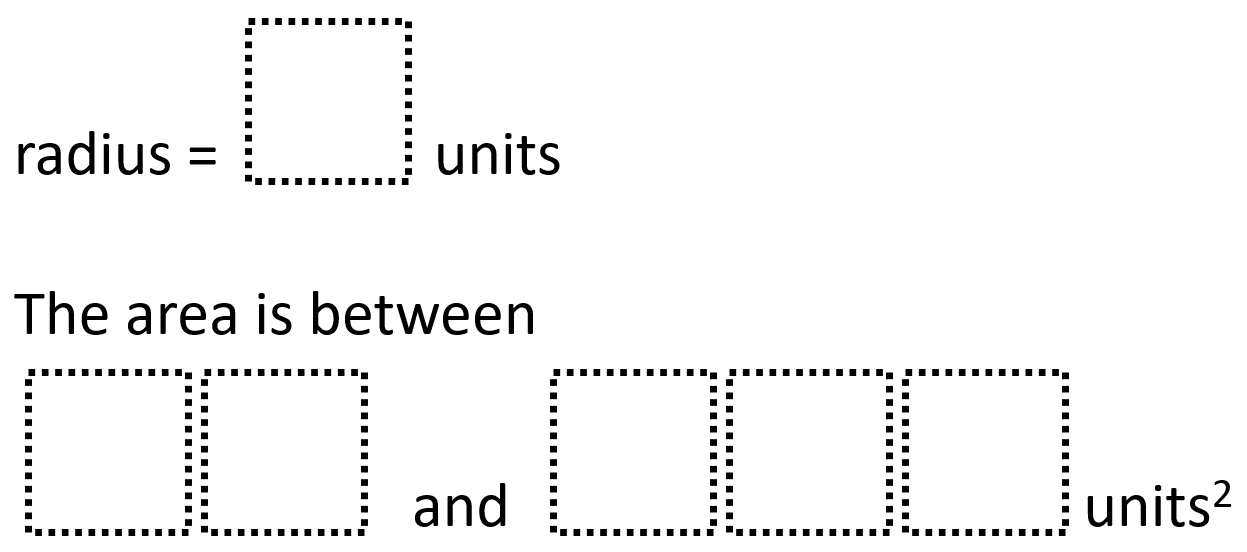# Circle Radius and Area 2

Directions: Using the digits 0 to 9 at most one time each, fill in the boxes to create a circle with the smallest difference between the area estimates.### Hint

How many digits of precision does pi require for this problem?

### Answer

The smallest difference between the area estimates is radius = 6 units and the area is between 98 and 123 square units

Source: Robert Kaplinsky

## Complimentary and Supplementary Angles 2

Directions: Using the digits 0 to 9 at most one time each, fill in the …